# Hyperfine Structure: Breit-Rabi Diagram

The energy-level shifts of a system with hyperfine structure in response to a magnetic field have a different character depending on whether the shifts are smaller or larger than the hyperfine splitting. When the Larmor frequency is much smaller than the hyperfine splitting, the hyperfine sublevels behave as if they were isolated, and the shift of each Zeeman sublevel is proportional to MF. For large magnetic fields, for which the Larmor frequency is larger than the hyperfine splitting, the hyperfine sublevels are strongly mixed with each other, and the system is best represented by the uncoupled |J MJI MI basis. The energy spectrum then consists of manifolds of levels with the same MJ and Zeeman shift proportional to MI. Here we analyze this situation for the ground state of 87Rb.

 Here is the label for the ground state of87Rb in the format used by the AtomicData database.
 Define the atomic system using values from the AtomicData database.
 The level diagram for the unperturbed system. For the diagrams, we use arbitrary units in which the hyperfine splitting is equal to 0.5.
 The Hamiltonian for the system subject to a z-directed magnetic field, with nominal Larmor frequency, whereis the Bohr magneton and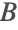is the magnetic-field strength.
 The level diagram showing diagonal shifts and hyperfine couplings induced by the magnetic field.
 Find the eigenvalues and eigenvectors of the Hamiltonian.
 Substitute in the numerical values of the hyperfine constant for 87Rb and the Bohr magneton.
 Rewrite the eigenenergies in GHz units, with the magnetic-field strength in kG.
 Plot the energies. This plot is known as a Breit-Rabi diagram.

The eigenvectors are the eigenstates in the presence of the magnetic field, written as a linear combination of the original sublevels. However, they are not listed in the order corresponding to the original sublevels. Here we reorder the eigenvalues and eigenvectors so that they match the unperturbed quantities when the magnetic field approaches zero.

 Normalize the eigenvectors and take the limit as the magnetic field goes to zero.
 Reorder the eigenvalues and eigenvectors to match the unperturbed eigenvalues and eigenvectors.

We can use the correctly ordered energies to plot the level diagram for the modified energy eigenstates in the presence of the magnetic field.

 Here is the level diagram with an adjustable magnetic field.

For a small field, the states are best described in the coupled basis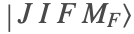, with three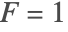sublevels (with), and five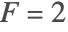sublevels (with). For a large field, the states are best described in the uncoupled basis |J MJI MI, with four MJ=1/2 states (with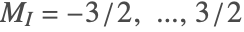), and four MJ=-1/2 states.